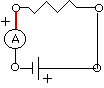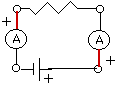Multi-meters current

It’s the same everywhere

Introduction

In a single loop of a circuit the electric current is the same everywhere.

Material

• A battery
• a bulb or resistor
• several (at least 2) multimeters

Assembly

See Making Simple Circuits for many techniques for connecting batteries and bulbs.

It’s easiest to use a battery holder, 2 clip leads, and a lamp holder.

To Do and Notice

Break the circuit between the battery and the bulb and insert a meter.Measure the current in the circuit. (Make sure you set the meter to the DC ampere scale. Always start on the scale that measures the largest currents then change the scale to more and more sensitive ranges until you get a reading.)

Break the circuit between the bulb and the battery (on the opposite side of the bulb) and insert a second meter.Measure the current on both meters. Notice that the currents are the same within the accuracy of each meter.

What’s Going On

In a single loop of a circuit such as this one, the electric current crossing any part of the circuit is the same.

This means that the current before and after the lamp is the same.

The lamp does not use up any of the current.

It also means that inside the lamp the current is the same and inside the battery the current is the same, although we cannot use our meters to measure these currents to confirm this statement.

Current flow can be modeled as the flow of positive charge around the circuit.

(Now in most circuits it is not the positive charge that flows. However, in most materials you don’t know what charge actually flows. Indeed, for almost all cases it doesn't matter whether the current is actually positives or negatives the result is the same. Since it is the same it is easiest to use the simplest model and just say it is the positives that actually flow.)

This is the conventional current flow model used by electrical engineers. In this model,the current flows from the positive terminal of the battery to the negative terminal, in the circuit outside the battery. Inside the battery the current continues to flow around the loop of the circuit in the same way. This means that the current of positives flows from the negative electrode to the positive electrode inside the battery.

Meters read positive current when the current flows into the plus terminal of the meter (The conventional current of positive charges). Usually the red lead is connected to the plus terminal. Notice how the (thicker) red leads of the meters are connected in the above diagrams.

Another model of current looks at the flow of negative charges. The negatively charged electrons are what actually flow in a coper wire. In this negative current model the electrons leave the negative terminal of the battery and flow through the bulb to the positive terminal of the battery. Inside the battery these mythical negative charges would flow from the positive terminal to the negative terminal. I say mythical charges because inside the battery electrolyte both positive and negative charges flow in opposite directions. In neither of these models do we need to know the exact details of the flow inside the battery, which is a good thing since it is complicated and confusing.

Etc

Scientists did not know the sign of the charge carrier in copper wires until 1916 with the results of the experiment of Tollman and Stewart. And yet, a great deal of correct electrical engineering was done without this knowledge.